Chapter Notes: Operations on Large Numbers

# Chapter Notes: Operations on Large Numbers - Notes | Study Mathematics for Class 5 - Class 5

 Table of contentsLarge NumbersArithmetic Operations on Large Numbers1. Addition2. Subtraction3. Multiplication4. DivisionOperations on Numbers using Estimation1 Crore+ students have signed up on EduRev. Have you?

Large Numbers

In a number system, the numbers that are ordinarily bigger or greater than the other numbers are known as large numbers. Large numbers are numbers that are more significant than the ones we use on a regular basis.

Example: 11 lakh, 11 million etc., come under the category of large numbers.

Arithmetic Operations on Large Numbers

The arithmetic operations on the large numbers are given below:

• Subtraction of Large Numbers
• Multiplication of Large Numbers
• Division of Large Numbers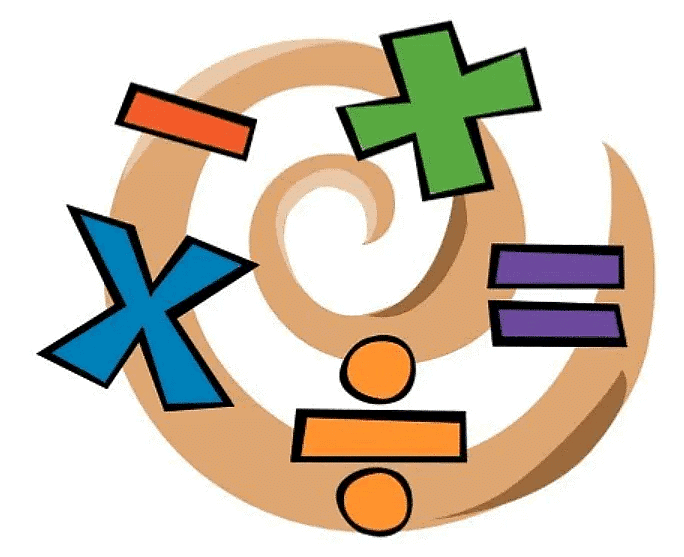Two or more numbers called the addends are added to give an answer called the sum. In addition of large numbers, you can place the numbers one below the other which you want to add so that one’s digit line in a column, ten’s digit line up in another column, and so on.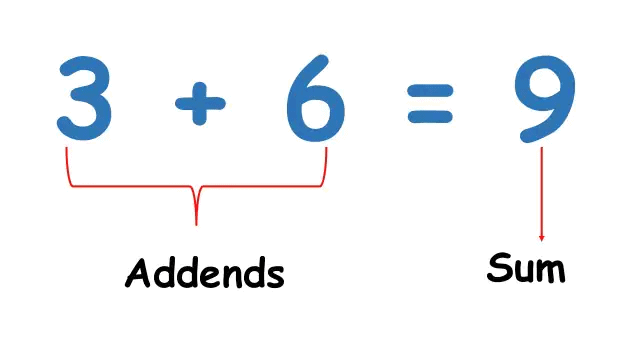Example 1: The statistics on the Internet suggest that the estimated population of Russia and Mexico is 14,39,64,709 and 13,07,59,074, respectively. If the population of the USA is 32,67,66,748, find out whether the population of Russia and Mexico taken together is more than that of the USA or not.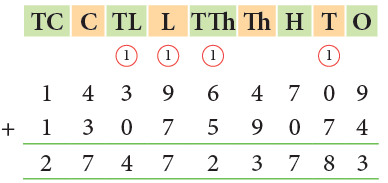Population of Russia and Mexico = 27,47,23,783
Population of USA = 32,67,66,748
Since, 3 ten crores > 2 ten crores, so the population of USA is more than the population of Russia and Mexico taken together.

Question for Chapter Notes: Operations on Large Numbers

2. Subtraction

When a number called subtrahend is subtracted from another number called minuend, the result is called their difference. The process is the same for the subtraction of large numbers. Instead of addition, you do the subtraction process, and it is called column subtraction.Example 2: The population of Greater Mumbai and Chennai as per the 2011 census was 1,63,68,084 and 64,24,624, respectively. How much more is Greater Mumbai’s population as compared to Chennai?

Population of Greater Mumbai = 1,63,68,084
Population of Chennai = 64,24,624
Population difference  = 1,63,68,084 – 64,24,624
= 99,43,460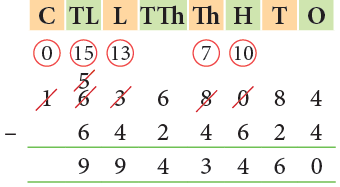Thus, population of Greater Mumbai is 99,43,460 more than that of Chennai.
You can check subtraction by adding the subtrahend to the difference. If the sum is equal to the minuend, then the subtraction has been carried out correctly.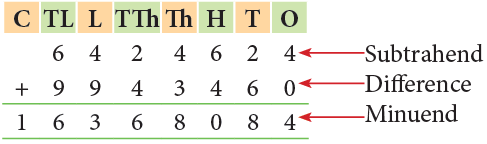Question for Chapter Notes: Operations on Large Numbers
Try yourself:Subtract 65248907 from 86165281

3. Multiplication

Multiplication is repeated addition. The number which is to be repeated or multiplied is called the multiplicand. The number which expresses how often the multiplicand is repeated is called the multiplier. In multiplying large numbers, you have to write down the numbers on top of each other and align the numbers.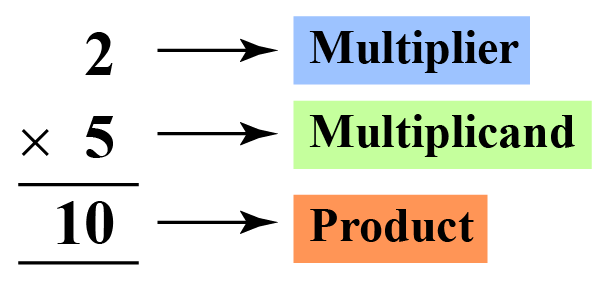Multiplier times the multiplicand = Product

For example: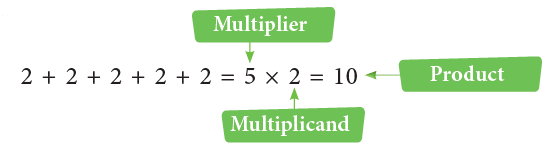• 2 added repeatedly five times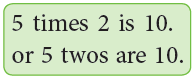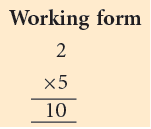The multiplier and the multiplicand are also called the factors.

Properties of Multiplication

1. Changing the order of factors does not change the product.
Example: 12 × 7 = 7 × 12 = 84
2. The product of any number and 1 is the number itself.
Example: 345 × 1 = 1 × 345 = 345
3. Changing the grouping of factors does not change the product.
Example: 2 × (4 × 9) = 2 × 36 = 72; (2 × 4) × 9 = 8 × 9 = 72
∴ 2 × (4 × 9) = (2 × 4) × 9 = 72.
4. The product of any number and 0 is 0.
Example: 79014 × 0 = 0

Multiplication by Multiples of 10

When one or both factors are multiples of 10, you can multiply mentally. Look at the following patterns to revise how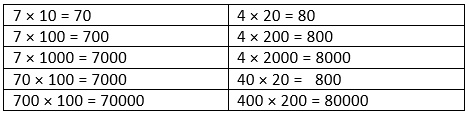• 7 × 1 ten = 7 tens = 70
4 × 2 hundreds = 8 hundreds
= 800
• To multiply by the multiples of 10, first find the product of the front non-zero digits. Then, write as many zeros after the product as there are zeros in both the factors.

Following the same pattern, we can mentally multiply a number by 10000 or 100000 and write the product.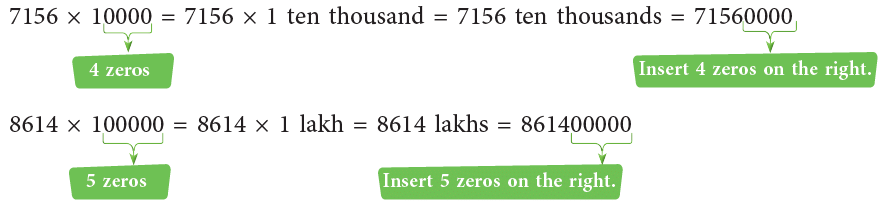Example 3: Find the products.
(a) 5078 × 200
(b) 1294 × 7000
(c) 71950 × 40000

(a) 5078 × 200 = 5078 × (2 × 100)
= (5078 × 2) × 100
= 10156 × 100 = 1015600
(b) 1294 × 7000 = 1294 × (7 × 1000)
= (1294 × 7) × 1000
= 9058 × 1000
= 9058000
(c) 71950 × 40000 = 71950 × (4 × 10000)
= (71950 × 4) × 10000
= 287800 × 10000
= 2878000000

Multiplying Large Numbers

Example 4: Multiply:
(a) 70935 by 36
(b) 32893 by 975

(a)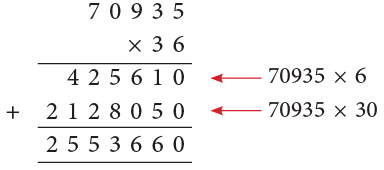∴ 70,935 × 36 = 25,53,660.

(b)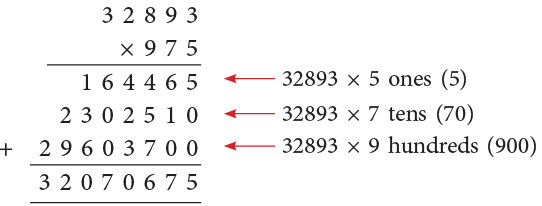∴ 32,893 × 975 = 3,20,70,675.

Example 5: Multiply 2892 by 9760.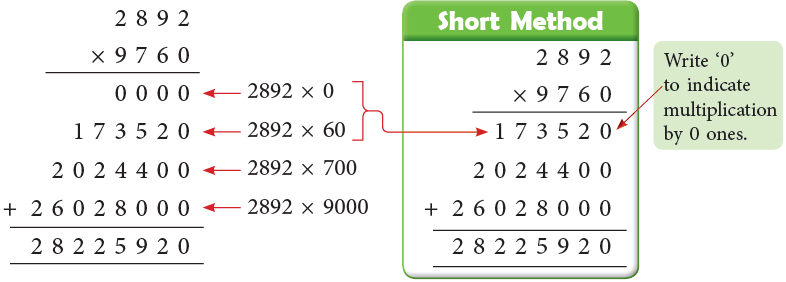∴ 2892 × 9760 = 2,82,25,920.

Question for Chapter Notes: Operations on Large Numbers
Try yourself:Multiply 39567 by 6094.

As shown below, if care is taken in placing the numerals, the zeros may be dropped without changing the product.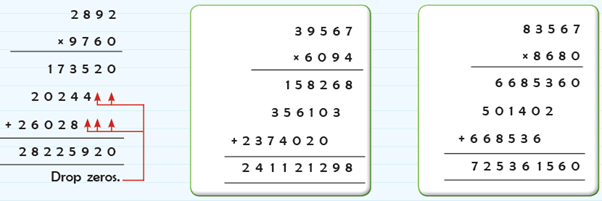4. Division

Division is the opposite of multiplication. The number to be divided is called the dividend. The number which divides is called the divisor and the result obtained is called the quotient. The number remaining, in the end, is called the remainder. For the division of large numbers, the long division method is used.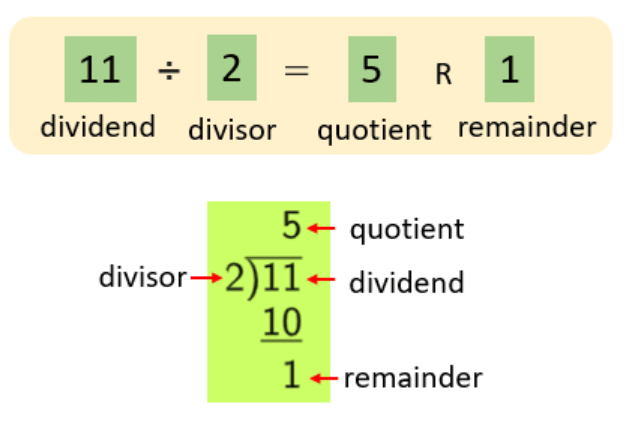Parts of a Division

Example 6: Divide 333 by 15.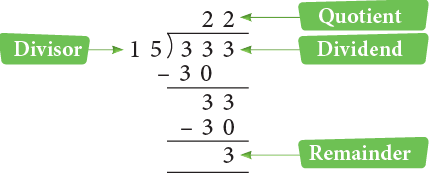∴ 333 ÷ 15 = 22 R 3
So, we can check division as under:
15 × 22 + 3 = 330 + 3 = 333

Properties of Division

1. Dividing a number by itself is equal to 1.
Example: 7876 ÷ 7876 = 1
2. Dividing zero by any number is equal to 0.
Example: 0 ÷ 38765 = 0
3. Dividing a number by 1 is equal to the number itself.
Example: 76208 ÷ 1 = 76208
4. Dividing any number by zero is not defined.
Example: 3893 ÷ 0 (not defined)

Division by Multiples of 10

You have studied about division by multiples of 10 in class 4 in detail. Let us revise the same here.
376 ÷ 10 ⇒ Quotient = 37; Remainder =   6
3594 ÷ 100 ⇒ Quotient = 35; Remainder =  94
7894 ÷ 1000 ⇒ Quotient =  7; Remainder = 894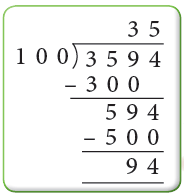Thus, we have,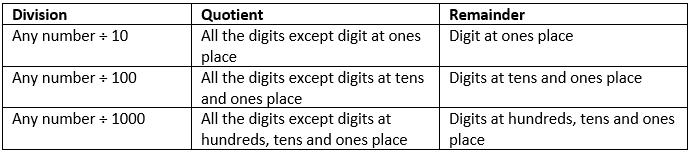Similarly,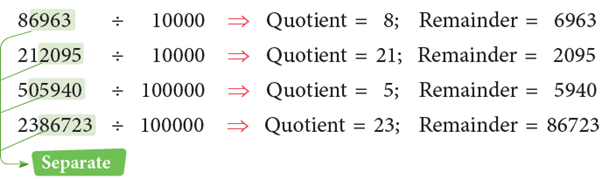Division by a 2-Digit Divisor

Example 7: Divide 6590898 by 95.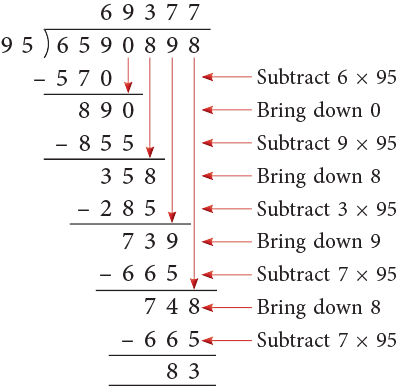∴ 6590898 ÷ 95 = 69377 R 83.
Take the first digit 9 of the divisor and divide 65 by 9. 9 sevens are 63 but 7 × 95 = 665, which is greater than 659. So, we take 6 in the quotient and subtract 6 × 95 = 570 from 659. Now, bring down 0. Take the first two digits of 890, i.e., 89. Divide by 9. 9 nines are 81 and 9 × 95 = 855. Subtract 855 from 890. Bring down 8. Now, proceed further in a similar manner till the remainder is less than 95.

Division by a 3-Digit Divisor

Example 8: Divide 371654 by 968.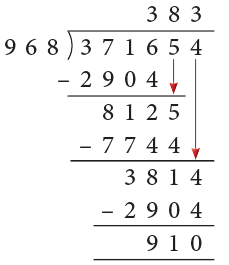∴ 371654 ÷ 968 = 383 R 910.
The divisor contains 3 digits, so we should consider 3 digits of the dividend, that is, 371. But since 371 is smaller than 968, we have to consider 4 digits, that is, 3716. Take the first digit 9 of the divisor and divide  37 by 9. The trial quotient is 4. But 4 × 968 gives 3872 which is greater than 3716, so take 3 as the quotient, 3 × 968 = 2904. Subtract 2904 from 3716 and bring down the next digit 5. Now, complete the division as shown.

Example 9: Divide 212588 by 697.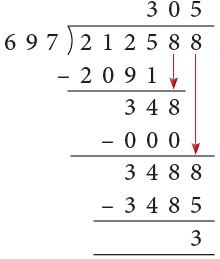∴ 212588 ÷ 697 = 305 R 3.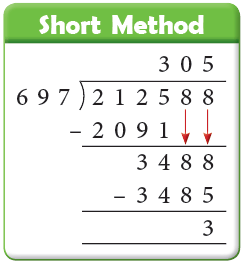We bring down 8 but the divisor 697 cannot divide 348 even once, so we put    0 after 3 in the quotient and bring down 8. Complete as shown.

Question for Chapter Notes: Operations on Large Numbers
Try yourself:Dividing 1886416 by 627. The Remainder we will get is:

Operations on Numbers using Estimation

• An estimate is a number close to an exact number which may be obtained by rounding off the exact number.
• We have already learned rounding off numbers in Chapter 3. It helps us to get to a number that is near the actual number.
• We can make use of rounding off the numbers to estimate the sums, differences, products, and quotients.

Estimating Sums

Example 10: The population of town X is 616817 and that of town Y is 293402. Estimate the total population of both towns to the nearest thousand.

Rounding off the numbers to the nearest thousand, we get,
616817 → 617000 and 293402 → 293000
∴ Estimated sum = 617000 + 293000 = 910000

Estimating Differences

Example 11: There were 67853 spectators in the stadium, out of which 15229 were children. Estimate the number of adults in the stadium to the nearest thousand.

Total number of spectators in the stadium = 68000 (Rounded off to the nearest thousand)
Total number of children in the stadium = 15000
∴ Number of adults in the stadium = 68000 – 15000 = 53000

Estimating Products

Example 12: A factory produces 19272 bulbs every month. About how many bulbs will be produced in a year? Also, find the actual answer.

Here, we need to find the product of number of bulbs produced in a month and the number of months.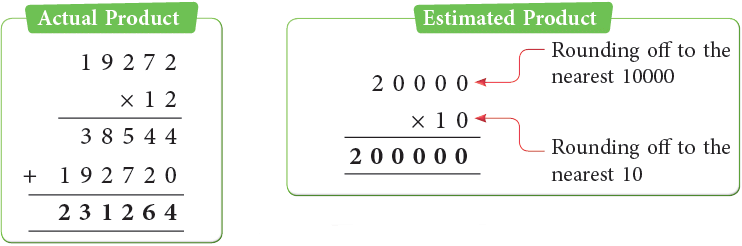While estimating an operation with two numbers with different number of digits, always round off the numbers to the highest place value.

Estimating Quotients

To estimate the quotient, we find the compatible numbers (a multiple of divisor). For example, to estimate the quotient of 72 and 432.

We round off 72 → 70 and 432 → 420.
∴ 7 × 6 = 42.
So, the estimated quotient is 6.

Example 13: The cost of 28 jackets is ₹ 41,580. Find the estimated cost of one jacket.

28 is rounded off to 30 and ₹ 41581 is rounded off to ₹ 42000 as 30 and 42000 are compatible numbers.
∴ Estimated quotient = 42000 ÷ 30 = 1400.
So, the estimated cost of one jacket is ₹ 1400.

The document Chapter Notes: Operations on Large Numbers - Notes | Study Mathematics for Class 5 - Class 5 is a part of the Class 5 Course Mathematics for Class 5.
All you need of Class 5 at this link: Class 5

## Mathematics for Class 5

12 videos|55 docs|35 tests
 Use Code STAYHOME200 and get INR 200 additional OFF

## Mathematics for Class 5

12 videos|55 docs|35 tests

Track your progress, build streaks, highlight & save important lessons and more!

,

,

,

,

,

,

,

,

,

,

,

,

,

,

,

,

,

,

,

,

,

;